Study P5 Mathematics Whole Numbers - Geniebook# Whole Numbers

The learning objectives are:

2. Multiplying or Dividing by 10, 100 or 1000 and their multiples

## 1. Reading And Writing Numbers

Whole numbers include zero and counting numbers. They are without fractions. In Primary 5, we will learn Numbers up to 10 million.

Let’s place the digits in 675 840 in their respective place values.We read 675 840 as six hundred and seventy-five thousand, eight hundred and forty.

The place value of the digit 6 is hundred thousands. The value of the digit 6 is 600 000.

The place value of the digit 7 is ten thousands. The value of the digit 7 is 70 000.

The place value of the digit 5 is thousands. The value of the digit 5 is 5000.

The place value of the digit 8 is hundreds. The value of the digit 8 is 800.

The place value of the digit 4 is tens. The value of the digit 4 is 40.

Remember the ‘s’ for the place values!

Let us try placing the digits in 9 675 840 in their respective place values.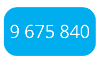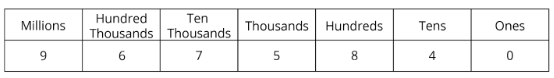We read 9 675 840 as nine million, six hundred and seventy-five thousand, eight hundred and forty.

The place value of the digit 9 is millions. The value of the digit 9 is 9 000 000.

It is a good habit to include spaces at the appropriate places when writing numbers in numerals.

Remember to include a comma and hyphen(s) at the appropriate places when writing numbers in words.

Question 1:

Write the following in numerals.Solution:

Place the digits in a place value chart as shown below.Three million, three hundred thousand, four hundred and thirty-five = 3 300 435

3 300 435

Question 2:

Write the following number in numerals.Solution:

Place the digits in a place value chart as shown below.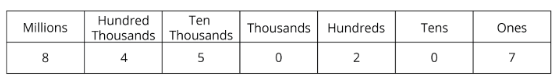8 450 207

Question 3:

Write the following in numerals.Solution:

Place the digits in a place value chart as shown below.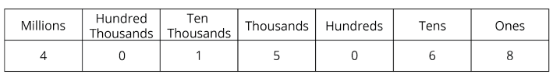4 015 068

Question 4:

Write the following in words.Solution:

Place the digits in a place value chart as shown below.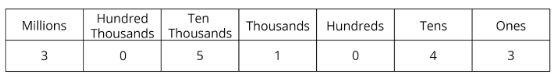3 051 043 = Three million, fifty-one thousand and forty-three

Three million, fifty-one thousand and forty-three

Question 5:

Write in words the following number.Solution:

Place the digits in a place value chart as shown below.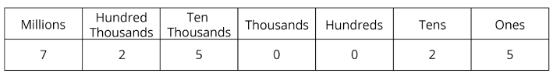7 250 025 = Seven million, two hundred and fifty thousand and twenty-five

Seven million, two hundred and fifty thousand and twenty-five

Question 6:

Write the following in words.Solution:

Place the digits in a place value chart as shown below.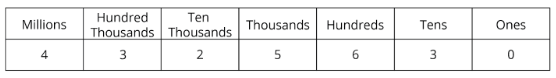4 325 630 = Four million, three hundred and twenty-five thousand, six hundred and thirty

Four million, three hundred and twenty-five thousand, six hundred and thirty

## 2. Multiplying or Dividing by 10, 100 or 1000 and their multiples

### Multiplying by 10, 100 or 1000

When we multiply a whole number by 10, we add one ‘0’ after the number.

Example:

5 × 10 = 50

When we multiply a whole number by 100, we add two ‘0’s after the number.

Example:

5 × 100 = 500

When we multiply a whole number by 1000, we add three ‘0’s after the number.

Example:

5 × 1000 = 5000

Question 1:

Match.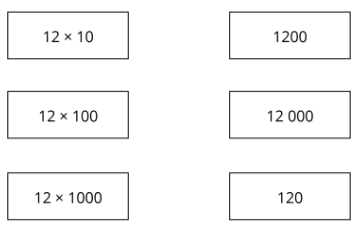Solution: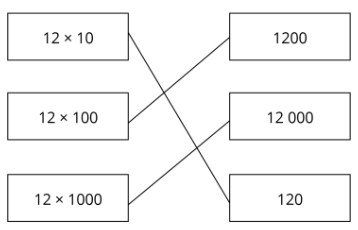### Multiplying by the multiples of 10, 100 or 1000

Now let’s multiply a number by the multiples of 10, 100 or 1000.

When we multiply a whole number by a multiple of 10, we express the multiple of 10 as a product of a number and 10.

Example:

12 × 50 = 12 × 5 × 10

= 60 × 10

= 600

When we multiply a whole number by a multiple of 100, we express the multiple of 100 as a product of a number and 100.

Example:

12 500 = 12 × 5 × 100

= 60 × 100

= 6000

When we multiply a whole number by a multiple of 1000, we express the multiple of 1000 as a product of a number and 1000.

Example:

12 5000 = 12 × 5 × 1000

= 60 × 1000

= 60 000

Question 1:

Do the following multiplication.

1. 27 × 1000 = __________
2. 27 × 5000 = __________

Solution:

1. 27 × 1000 = 27 000

1. 27 × 5000 = 27 × 5 × 1000

= 135 × 1000

= 135 000

1. 27 000
2. 135 000

Question 2:

Do the following multiplication.

319 × 6000 = _________

Solution:

319 6000 = 319 × 6 × 1000

= 1 914 000

1 914 000

Question 3:

Do the following multiplication.

700 × 9000 = _________

Solution:

7 × 100 × 9 × 1000 = 7 × 9 × 100 × 1000

= 6 300 000

6 300 000

Question 4:

Fill in the blanks.

1. 12 × __________ = 12 000
2. 12 × __________ = 120 000

Solution:

1. 12 ×    1000    = 12 000
2. 12 ×  10 000   = 120 000

1. 1000
2. 10 000

Question 5:

Fill in the blanks

60 × __________ = 720

Solution:

60 × ________ = 720

60 ×       12       = 720

12

### Dividing by 10, 100 or 1000

When we divide a whole number by 10, we remove one ‘0’ after the number.

Example

70 000 ÷ 10 = 7000

When we divide a whole number by 100, we remove two ‘0’s after the number.

Example:

70 000 ÷ 100 = 700

When we divide a whole number by 1000, we remove three ‘0’s after the number.

Example:

70 000 ÷ 1000 = 70

Question 1:

Do the following division.

880 ÷ 10 = __________

Solution:

880 ÷ 10 = 88

88

Question 2:

Do the following division.

293 000 ÷ 100 = __________

Solution:

293 000 ÷ 100 = 2930

2930

Question 3:

Do the following division.

630 000 ÷ 1000 =__________

Solution:

630 000 ÷ 1000 = 630

630

## Dividing by the multiples of 10, 100 or 1000

Now let’s divide a number by the multiples of 10, 100 or 1000.

When we divide a whole number by a multiple of 10, we break the multiple of 10 into 10 and a number. We will divide by 10 first, followed by the number.

Example:

30 000 ÷ 60 = 30 000 ÷ 10 ÷ 6

= 3000 ÷ 6

= 500

When we divide a whole number by a multiple of 100, we break the multiple of 100 into 100 and a number. We will divide by 100 first, followed by the number.

Example:

30 000 ÷ 600 = 30 000 ÷ 100 ÷ 6

= 300 ÷ 6

= 50

When we divide a whole number by a multiple of 1000, we break the multiple of 1000 into 1000 and a number. We will divide by 1000 first, followed by the number.

Example:

30 000 6000 = 30 000 ÷ 1000 ÷ 6

= 30 ÷ 6

= 5

Question 1:

Do the following division.

36 600 ÷ 60 =__________

Solution:

36 600 ÷ 60 = 36 600 ÷ 10 ÷ 6

= 3660 ÷ 6

= 610

610

Question 2:

Do the following division.

44 800 ÷ 800 =__________

Solution:

44 800 ÷ 800 = 44 800 ÷ 100 ÷ 8

= 448 ÷ 8

= 56

56

Question 3:

Do the following division.

630 000 ÷ 9000 =__________

Solution:

630 000 ÷ 9000 = 630 000 ÷ 1000 ÷ 9

= 630 ÷ 9

= 70

70

Question 4:

Fill in the blanks with the correct answer.

8400 ÷ __________= 70

Solution:

8400 ÷ 70 = 8400 ÷ 10 ÷ 7

= 840 ÷ 7

= 120

120

## Conclusion

In this article, we learnt about the Whole Numbers as per the Primary 5 Math level. We learnt the following subtopics in Whole Numbers:

• Reading and Writing Numbers up to 10 million
• Multiplication and Division by 10, 100, or 1000 and their multiples.

Continue Learning
Volume Of A Liquid Decimals - Operations & Conversions
Ratio: Introduction Average - Formula
Percentage, Fractions And Decimals Whole Numbers
Strategy - Equal Stage Angle Properties
Table Rates Whole Number Strategy: Gap & Difference
Fractions - Addition & Subtraction Ratio Strategy: Repeated IdentityPrimaryPrimary 1Primary 2Primary 3Primary 4Primary 5EnglishMaths
Volume Of A Liquid
Decimals - Operations & Conversions
Ratio: Introduction
Average - Formula
Percentage, Fractions And Decimals
Whole Numbers
Strategy - Equal Stage
Angle Properties
Table Rates
Whole Number Strategy: Gap & Difference
Ratio Strategy: Repeated IdentitySciencePrimary 6SecondarySecondary 1Secondary 2Secondary 3Secondary 4